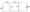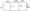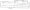# CSVTU Exam Papers – BE I Year – Basic Electrical Engineering – May-June 2007

BE ( I year )

Examination May-June 2007

Basic Electrical Engineering

Q1 (a)  Find current through branch a b in the circuit shown in fig – by using loop current method and superposition method. not change the sources.(b)Find current through branch a b of circuit shown in fig 2 using  Norton’s Theorem.(c) A series magnetic circuit has an iron path of length 50 and an air gap length of 1 mm. The cross sectional ares iron is 6 cm2 and coil would on iron has  400 turns Determine the current required to produce a flux in circuit.The points on magnetization curve of iro

Flux density     wb/m2            1.2                        1.35                              1.55                        1.55

H (a tm)                                           500                       1000                            2000                     4500

Q2(a) A 20 Ohm  resistance is connected in series with inductor and  capacitance across a 25 v variable frequency supply when the frequency is 400 Hz , The currents in circuit is at its maximum,”value of 0.5 A and potential difference ac roses capacitor b 150 V. Find C of capacitor and r & L  of inductor Draw pharos diagram representing voltage across each element and current.

(b)In the circuit shown in fig.-3 find I,VI & V2 The value of  impedances shown are in ohms. Represent them diagram . Take supply V= 99 √2 volts (RMS)(c)A three phase star connected balance load of value z=4060 ohms per phase is connected across 400 v, 3- phase balance supply. Find power drawn from supply from and reading of two wattmeter’s connected to measure the input . Draw circuit diagram .

Q3(a) How will you measure has primary’and secondary turns of 100 and 80 respectively . The resistance and leakage reactance of primary winding is 0.3 Ohm and 1.1 Ohm  and that of secondary winding is 0.01 Ohm  and 0.035  Ohm  respectively. The primary voltage is 2000 V. Calculate full load regulation at 0.8 puff, and lead. At what power factor regulation is zero.

(b)In a transformer the hysteretic and eddy current loss was found to be ph = 300W and per = 250W at 400V, 50Hz supply. Find the iron loss at800V, 100Hz.

Q4(a) A4 pole dc shunt motor takes 22A from 220A from 220V supply. The armature and field resistances are and . The armature is lap connected with 300 conductors if flux per pole is 20m Web. Calculate speed and gross torque developed.

(b) A three phase induction motor runs at 290 rpm at full load with 5o Hz supply. Z and number of poles motor and slip.

(c)Draw the circuit diagrams of following motors-

(i) D.C. shunt motor

(ii) D.C. Series motor

(iii) Three phase slipping induction motor

(iv) Single phase capacitor start induction motor

Give applications of above motors along with brief explanation

Q5 (a) Describe the construction and working principles of any one type of wattmeter.

(b)Why is damping torques provided in a electrical instrument ? What are different methods of providing force ? Describe them what lype of damping is provided in following instruments ?

(i)  PMMC

(ii) Moving coil

(iii) Moving iron

(iv) Wattmeter

(c)  A moving coil instrument has resistance of 10 and gives foil scale deflection when carrying a current of 50mA. Show how it can be adopted to measure voltage unto 750V and current unto 100 amp.